Skip to main content Accessibility help
Home
Hostname: page-component-564cf476b6-pxp6n Total loading time: 0.235 Render date: 2021-06-22T11:07:45.499Z Has data issue: true Feature Flags: { "shouldUseShareProductTool": true, "shouldUseHypothesis": true, "isUnsiloEnabled": true, "metricsAbstractViews": false, "figures": true, "newCiteModal": false, "newCitedByModal": true, "newEcommerce": true }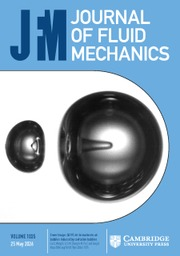Journal of Fluid Mechanics

# Bifurcation analysis of steady natural convection in a tilted cubical cavity with adiabatic sidewalls

Published online by Cambridge University Press:  04 September 2014

Corresponding
E-mail address:

## Abstract

Natural convection in an inclined cubical cavity heated from two opposite walls maintained at different temperatures and with adiabatic sidewalls is investigated numerically. The cavity is inclined by an angle$\def \xmlpi #1{}\def \mathsfbi #1{\boldsymbol {\mathsf {#1}}}\let \le =\leqslant \let \leq =\leqslant \let \ge =\geqslant \let \geq =\geqslant \def \Pr {\mathit {Pr}}\def \Fr {\mathit {Fr}}\def \Rey {\mathit {Re}}\theta$ around a lower horizontal edge and the isothermal wall set at the higher temperature is the lower wall in the horizontal situation (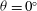$\theta = 0^\circ$ ). A continuation method developed from a three-dimensional spectral finite-element code is applied to determine the bifurcation diagrams for steady flow solutions. The numerical technique is used to study the influence of${\theta }$ on the stability of the flow for moderate Rayleigh numbers in the range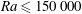$\mathit{Ra} \leq 150\, 000$ , focusing on the Prandtl number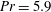$\mathit{Pr} = 5.9$ . The results show that the inclination breaks the degeneracy of the stable solutions obtained at the first bifurcation point in the horizontal cubic cavity: (i) the transverse stable rolls, whose rotation vector is in the same direction as the inclination vector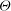${\boldsymbol{\Theta}}$ , start from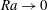$\mathit{Ra} \to 0$ forming a leading branch and becoming more predominant with increasing$\theta$ ; (ii) a disconnected branch consisting of transverse rolls, whose rotation vector is opposite to${\boldsymbol{\Theta}}$ , develops from a saddle-node bifurcation, is stabilized at a pitchfork bifurcation, but globally disappears at a critical inclination angle; (iii) the semi-transverse stable rolls, whose rotation axis is perpendicular to${\boldsymbol{\Theta}}$ at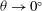$\theta \to 0^\circ$ , develop from another saddle-node bifurcation, but the branch also disappears at a critical angle. We also found the stability thresholds for the stable diagonal-roll and four-roll solutions, which increase with$\theta$ until they disappear at other critical angles. Finally, the families of stable solutions are presented in the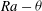$\mathit{Ra}-\theta$ parameter space by determining the locus of the primary, secondary, saddle-node, and Hopf bifurcation points as a function of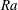$\mathit{Ra}$ and$\theta$ .

## JFM classification

Type
Papers
Information
Journal of Fluid Mechanics , 10 October 2014 , pp. 650 - 688
Copyright
© 2014 Cambridge University Press

## Access options

Get access to the full version of this content by using one of the access options below.

## References

Adachi, T. 2006 Stability of natural convection in an inclined square duct with perfectly conducting side walls. Intl J. Heat Mass Transfer 49, 23722380.CrossRefGoogle Scholar
Altaç, Z. & Kurtul, Ö. 2007 Natural convection in tilted rectangular enclosures with a vertically situated hot plate inside. Appl. Therm. Engng 27, 18321840.CrossRefGoogle Scholar
Baumgardner, J. R. 1985 Three-dimensional treatment of convective flow in the Earth’s mantle. J. Stat. Phys. 39, 501511.CrossRefGoogle Scholar
Beaume, C., Bergeon, A. & Knobloch, E. 2013 Convectons and secondary snaking in three-dimensional natural doubly diffusive convection. Phys. Fluids 25, 024105.CrossRefGoogle Scholar
Bénard, H. 1901 Les tourbillons cellulaires dans une nappe liquide. – Méthodes optiques d’observation et d’enregistrement. J. Phys. Theor. Appl. 10, 254266.CrossRefGoogle Scholar
Ben Hadid, H. & Henry, D. 1997 Numerical study of convection in the horizontal Bridgman configuration under the action of a constant magnetic field. Part 2: three-dimensional flow. J. Fluid Mech. 333, 5783.CrossRefGoogle Scholar
Bergeon, A., Ghorayeb, K. & Mojtabi, A. 1999 Double diffusive instability in an inclined cavity. Phys. Fluids 11, 549559.CrossRefGoogle Scholar
Bergeon, A., Henry, D., Ben Hadid, H. & Tuckerman, L. S. 1998 Marangoni convection in binary mixtures with Soret effect. J. Fluid Mech. 375, 143177.CrossRefGoogle Scholar
Bergeon, A., Henry, D. & Knobloch, E. 2001 Three-dimensional Marangoni–Bénard flows in square and nearly square containers. Phys. Fluids 13, 9298.CrossRefGoogle Scholar
Bergeon, A. & Knobloch, E. 2002 Natural doubly diffusive convection in three-dimensional enclosures. Phys. Fluids 14, 32333250.CrossRefGoogle Scholar
Borońska, K. & Tuckerman, L. S. 2010 Extreme multiplicity in cylindrical Rayleigh–Bénard convection. II. Bifurcation diagram and symmetry classification. Phys. Rev. E 81, 036321.Google ScholarPubMed
Catton, I. 1972 The effect of insulating vertical walls on the onset of motion in a fluid heated from below. Intl J. Heat Mass Transfer 15, 665672.CrossRefGoogle Scholar
Cliffe, K. A. & Winters, K. H. 1984 A numerical study of the cusp catastrophe for Bénard convection in tilted cavities. J. Comput. Phys. 54, 531534.CrossRefGoogle Scholar
Cliffe, K. A. & Winters, K. H. 1986 The use of symmetry in bifurcation calculations and its application to the Bénard problem. J. Comput. Phys. 67, 310326.CrossRefGoogle Scholar
Emanuel, K. A. 1994 Atmospheric Convection. Oxford University Press.Google Scholar
Fusegi, T., Hyun, J. M., Kuwahara, K. & Farouk, B. 1991 A numerical study of three-dimensional natural convection in a differentially heated cubical enclosure. Intl J. Heat Mass Transfer 34, 15431557.CrossRefGoogle Scholar
Getling, A. V. 1998 Rayleigh–Bénard Convection: Structures and Dynamics. World Scientific.CrossRefGoogle Scholar
Henry, D. & Ben Hadid, H. 2007 Multiple flow transitions in a box heated from the side in low-Prandtl-number fluids. Phys. Rev. E 76, 016314.CrossRefGoogle Scholar
Juel, A., Mullin, T., Ben Hadid, H. & Henry, D. 2001 Three-dimensional free convection in molten gallium. J. Fluid Mech. 436, 267281.CrossRefGoogle Scholar
Karniadakis, G. E., Israeli, M. & Orszag, S. A. 1991 High-order splitting method for the incompressible Navier–Stokes equations. J. Comput. Phys. 97, 414443.CrossRefGoogle Scholar
Kirchartz, K. R. & Oertel, H. Jr 1988 Three-dimensional thermal cellular convection in rectangular boxes. J. Fluid Mech. 192, 249286.CrossRefGoogle Scholar
Koutsoheras, W. & Charters, W. W. S. 1977 Natural convection phenomena in inclined cells with finite side-walls – a numerical solution. Solar Energy 19, 433438.CrossRefGoogle Scholar
Lappa, M. 2005 Thermal convection and related instabilities in models of crystal growth from the melt on earth and in microgravity: past history and current status. Cryst. Res. Technol. 40, 531549.CrossRefGoogle Scholar
Lo Jacono, D., Bergeon, A. & Knobloch, E. 2013 Three-dimensional spatially localized binary-fluid convection in a porous medium. J. Fluid Mech. 730, R2.CrossRefGoogle Scholar
Mallinson, G. D. & Davis, G. D. V. 1977 Thee-dimensional natural convection in a box: a numerical study. J. Fluid Mech. 83, 131.CrossRefGoogle Scholar
Mamun, C. K. & Tuckerman, L. S. 1995 Asymmetry and Hopf bifurcation in spherical Couette flow. Phys. Fluids 7, 8091.CrossRefGoogle Scholar
Mercader, I., Batiste, O. & Alonso, A. 2010 An efficient spectral code for incompressible flows in cylindrical geometries. Comput. Fluids 39, 215224.CrossRefGoogle Scholar
Mohamad, A. A., Sicard, J. & Bennacer, R. 2006 Natural convection in enclosures with floor cooling subjected to a heated vertical wall. Intl J. Heat Mass Transfer 49, 108121.CrossRefGoogle Scholar
Moya, S. L., Ramos, E. & Sen, M. 1987 Numerical study of natural convection in a tilted rectangular porous material. Intl J. Heat Mass Transfer 30, 741756.CrossRefGoogle Scholar
Ozoe, H., Fujii, K., Noam, L. & Churchill, S. W. 1983 Long rolls generated by natural convection in an inclined, rectangular enclosure. Intl J. Heat Mass Transfer 26, 14271438.CrossRefGoogle Scholar
Ozoe, H., Sayama, H. & Churchill, S. W. 1974 Natural convection in an inclined square channel. Intl J. Heat Mass Transfer 17, 401406.Google Scholar
Ozoe, H., Sayama, H. & Churchill, S. W. 1977 Natural convection patterns in a long inclined rectangular box heated from below: Part I. Three-directional photography. Intl J. Heat Mass Transfer 20, 123129.CrossRefGoogle Scholar
Pallares, J., Arroyo, M. P., Grau, F. X. & Giralt, F. 2001 Experimental laminar Rayleigh–Bénard convection in a cubical cavity at moderate Rayleigh and Prandtl numbers. Exp. Fluids 31, 208218.CrossRefGoogle Scholar
Petrone, G., Chénier, E. & Lauriat, G. 2004 Stability of free convection in air-filled horizontal annuli: influence of the radius ratio. Intl J. Heat Mass Transfer 47, 38893907.CrossRefGoogle Scholar
Puigjaner, D., Herrero, J., Giralt, F. & Simó, C. 2004 Stability analysis of the flow in a cubical cavity heated from below. Phys. Fluids 16, 36393655.CrossRefGoogle Scholar
Puigjaner, D., Herrero, J., Giralt, F. & Simó, C. 2006 Bifurcation analysis of multiple steady flow patterns for Rayleigh–Bénard convection in a cubical cavity at Pr = 130. Phys. Rev. E 73, 046304.CrossRefGoogle Scholar
Puigjaner, D., Herrero, J., Simó, C. & Giralt, F. 2008 Bifurcation analysis of steady Rayleigh–Bénard convection in a cubical cavity with conducting sidewalls. J. Fluid Mech. 598, 393427.CrossRefGoogle Scholar
Rayleigh, L. 1916 On convective currents in a horizontal layer of fluid when the higher temperature is on the under side. Phil. Mag. 32 (192), 529546.CrossRefGoogle Scholar
Seydel, R. 2009 Practical Bifurcation and Stability Analysis. Springer.Google Scholar
Torres, J. F., Henry, D., Komiya, A., Maruyama, S. & Ben Hadid, H. 2013 Three-dimensional continuation study of convection in a tilted rectangular enclosure. Phys. Rev. E 88, 043015.CrossRefGoogle Scholar
Wakashima, S. & Saitoh, T. S. 2004 Benchmark solutions for natural convection in a cubic cavity using the high-order time–space method. Intl J. Heat Mass Transfer 47, 853864.CrossRefGoogle Scholar
18
Cited by

# Send article to Kindle

To send this article to your Kindle, first ensure no-reply@cambridge.org is added to your Approved Personal Document E-mail List under your Personal Document Settings on the Manage Your Content and Devices page of your Amazon account. Then enter the ‘name’ part of your Kindle email address below. Find out more about sending to your Kindle. Find out more about sending to your Kindle.

Note you can select to send to either the @free.kindle.com or @kindle.com variations. ‘@free.kindle.com’ emails are free but can only be sent to your device when it is connected to wi-fi. ‘@kindle.com’ emails can be delivered even when you are not connected to wi-fi, but note that service fees apply.

Find out more about the Kindle Personal Document Service.

Bifurcation analysis of steady natural convection in a tilted cubical cavity with adiabatic sidewalls
Available formats
×

# Send article to Dropbox

To send this article to your Dropbox account, please select one or more formats and confirm that you agree to abide by our usage policies. If this is the first time you use this feature, you will be asked to authorise Cambridge Core to connect with your <service> account. Find out more about sending content to Dropbox.

Bifurcation analysis of steady natural convection in a tilted cubical cavity with adiabatic sidewalls
Available formats
×

# Send article to Google Drive

To send this article to your Google Drive account, please select one or more formats and confirm that you agree to abide by our usage policies. If this is the first time you use this feature, you will be asked to authorise Cambridge Core to connect with your <service> account. Find out more about sending content to Google Drive.

Bifurcation analysis of steady natural convection in a tilted cubical cavity with adiabatic sidewalls
Available formats
×
×

#### Reply to:Submit a response

Please enter your response.

#### Your details

Please enter a valid email address.

#### Conflicting interests

Do you have any conflicting interests? *